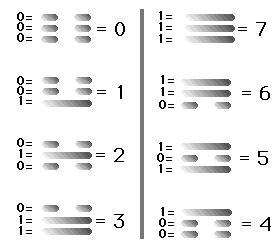## Wednesday, June 11, 2003

### The I Ching as a Binary System (易經二進制)

The I Ching as a Binary System (易經二進制)
[通訊技術 ]
(2003/06/11)I Ching as a Binary System

Let us, as did Leibniz in the 16th century, look at the Yin and Yang symbols as binary notation. Binary means "in twos." The Yin and Yang symbols are a way of counting in twos.

We normally use decimal notation. Decimal means "in tens." The ten counting symbols we use are

0, 1, 2, 3, 4, 5, 6, 7, 8 and 9. After 9 we continue counting by doubling the numbers: 10, 11, 12, 13,

The same principle applies in binary arithmetic. The two numbers used in binary arithmetic are 0 and 1.

1, 10, 11, 100, 101, 110, 111, 1000, 1001, is the way one counts from 0 to 9.

Linking the decimal and binary notations we find that 0 = 0, 1=1, 2=10, 3=11, 4=100, 5=101, 6=110, 7=111, just as

000, 001, 002, 003, 004, 005, 006, 007, means the same as 0, 1, 2, 3, 4, 5, 6, 7. (The zeros to the left are there so that we have three digits in each decimal group.)

So also does 000, 001, 010, 011, 100, 101, 110, 111, mean the same as 0, 1, 10, 11, 100, 101, 110, 111. (The zeros to the left are there so that we have three digits in each binary group.)

So putting the decimal and binary sequence together:

0 = 000
1 = 001
2 = 010
3 = 011
4 = 100
5 = 101
6 = 110
7 = 111

The sequence 000, 001, 010, 100, 101, 110, 111 gives us the link to the Trigrams. With this link we can discover the number value of each Trigram.

We write horizontally. The Chinese write vertically. So the first thing we do with the

000, 001, 010, 100, 101, 110, 111 sequence

is to stack the numbers on top of each other instead of side-by-side.

0 0 0 0 1 1 1 1
0 0 1 1 0 0 1 1
0 1 0 1 0 1 0 1

Notice how the Yang energy, represented by the 1's, rises from the lower to the upper positions when viewed as a sequence flowing from left to right.

The next step is to substitute a Yin line for 0 and a Yang line for 1 and Bingo! -- we have constructed our eight Trigrams.

Discovering the connection between the binary code and the I Ching leads to connections with other organizing patterns such as the 64 codons of the genetic code as well as the pattern of the table of elements.

Anyone who owns a PC as of the year 2003, even ordinary end users who do not qualify as either hardware or software experts, will recognize certain recurring numbers in both hardware and software specifications. Among these numbers are 1, 2, 4, 8, 16, 32, 64, 128, 256, 512, 1024, and so on. In the case of hardware for example, my personal PC has 256MB inline memory modules. In the case of software, my personal PC uses Windows XP Pro, which is a 32-bit Operating System.

These numbers recur constantly in the world of computing for the simple reason that they are the binary equivalent of "round numbers." They are the binary counterparts of 1, 10, 100, 1000, 10,000, and so on in the decimal system. To anyone with even a nodding acquaintance with the "8 Trigrams" or "64 Hexagrams" of the I Ching, these numbers will seem like old friends.

-- Bevin Chu

Explanation: I Ching as Binary System
Illustration(s): The Eight Trigrams
Author(s): Roderic Sorrell and Amy Max Sorrell
Affiliation: "An Idiot's Guide to the I Ching" Published by Bio-Ching Publishing
Source: http://bioching.com/iching/
Publication Date: 1992
Original Language: English
Editor: Bevin Chu, Registered Architect

Unknown said...

Very interesting and intriguing! Thanks for sharing.

Bevin Chu said...

Dear Doc Stier,

Thanks!

Be sure to check out

The Digital Revolution began with Fu Xi

http://proporzionedivina.blogspot.tw/2009/06/digital-revolution-began-with-fu-xi.html

It puts the ubiquitous allegations that "The Chinese only know how to steal technology secrets from the West" in perspective.